Home | | Theory of Computation | Grammars - Regular Expressions and Languages

# Grammars - Regular Expressions and Languages

1. Grammar Introduction 2. Types of Grammar 3. Context Free Grammars and Languages 4. Derivations and Languages 5. Ambiguity 6. Relationship between derivation and derivation trees 7. Simplification of CFG 8. Elimination of Useless symbols 9. Unit productions 10. Null Productions 11. Greibach Normal Form Chomsky normal form 12. Problems related to CNF and GNF

GRAMMARS

Regular Expressions: Formal

We construct REs from primitive constituents (basic elements) by repeatedly applying certain recursive rules as given below. (In the definition)

Definition : Let S be an alphabet. The regular expressions are defined recursively as

Basis :These are called primitive regular expression i.e. Primitive

Recursive Step :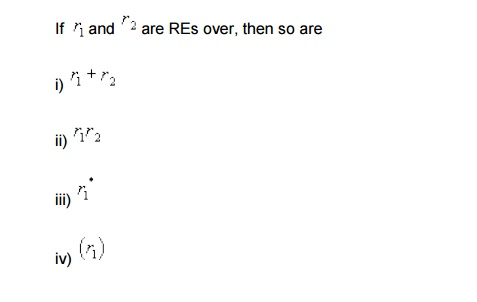Closure : r is RE over only if it can be obtained from the basis elements (Primitive REs) by a finite no of applications of the recursive step (given in 2).

Example : Let  å = { 0,1,2 }. Then (0+21)*(1+ F ) is a RE, because we can construct this expression applying the above rules as given in the following step.Language described by REs :  Each describes a language (or a language is associated with every RE).

Notation : If r is a RE over some alphabet then L(r) is the language associate with r . We can define theExample : Consider the RE (0*(0+1)). Thus the language denoted by the RE is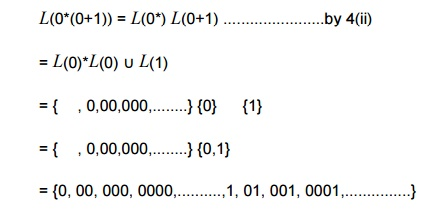Precedence Rule

Consider the RE ab + c. The language described by the RE can be thought of either L(a)L(b+c) or L(ab)È L( c) as provided by the rules (of languages described by REs) given already. But these represents two different languages lending to ambiguity. To remove this ambiguity we can either

1)      Use fully parenthesized expression- (cumbersome) or

2)      Use a set of precedence rules to evaluate the options of REs in some order. Like other algebras mod in mathematics.

For REs, the order of precedence for the operators is as follows:

i)       The star operator precedes concatenation and concatenation precedes union (+) operator.

ii)      It is also important to note that concatenation & union (+) operators are associative and union operation is commutative.

Using these precedence rule, we find that the RE ab+c represents the language L(ab) È L(c) i.e. it should be grouped as ((ab)+c).

We can, of course change the order of precedence by using parentheses. For example, the language represented by the RE a(b+c) is L(a)L(b+c).

Example : The RE ab*+b is grouped as ((a(b*))+b) which describes the language L(a)(L(b))* ÈL(b)

Example : The RE (ab)*+b represents the language (L(a)L(b))* È L(b).

Example : It is easy to see that the RE (0+1)*(0+11) represents the language of all strings over {0,1} which are either ended with 0 or 11.

Example : The regular expression r =(00)*(11)*1 denotes the set of all strings with an even number of 0's followed by an odd number of 1's i.e.

Note : The notation  r+ is used to represent the RE rr*. Similarly, r2 represents the RE rr,r3  denotes r2r, and so on.

An arbitrary string over å = {0,1} is denoted as (0+1)*.

Exercise : Give a RE r over {0,1} s.t.

L(r)=has at least one pair of consecutive 1's}

Solution : Every string in L(r) must contain 00 somewhere, but what comes before and what goes before is completely arbitrary. Considering these observations we can write the REs as (0+1)*11(0+1)*.

Example : Considering the above example it becomes clean that the RE (0+1)*11(0+1)*+(0+1)*00(0+1)* represents the set of string over {0,1} that contains the substring 11 or 00.

Example : Consider the RE 0*10*10*. It is not difficult to see that this RE describes the set of strings over {0,1} that contains exactly two 1's. The presence of two 1's in the RE and any no of 0's before, between and after .

Example : Consider the language of strings over {0,1} containing two or more

Solution : There must be at least two 1's in the RE somewhere and what comes before, between, and after is completely arbitrary. Hence we can write the RE as (0+1)*1(0+1)*1(0+1)*. But following two REs also represent the same language, each ensuring presence of least two 1's somewhere in the string

i) 0*10*1(0+1)*

ii) (0+1)*10*10*

Example : Consider a RE r over {0,1} such thathas no pair of consecutive 1's}

Solution : Though it looks similar to ex ……., it is harder to construct to construct. We observer that, whenever

a 1 occurs, it must be immediately followed by a 0. This substring may be preceded & followed by any no of 0's. So the final RE must be a repetition of strings of the form: 00…0100….00 i.e. 0*100*. So it looks like the these observations into consideration, the final RE is r = (0*100*)(1+ Î )+0*(1+ Î).

Alternative Solution :

The language can be viewed as repetitions of the strings 0 and 01. Hence get the RE as r = (0+10)*(1+ Î).This is a shorter expression but represents the same language.

Regular Expression:

FA to regular expressions:

FA to RE (REs for Regular Languages)

Lemma: If a language is regular, then there is a RE to describe it. i.e. if L = L(M) for some DFA M, then there is a RE r such that L = L(r).

Proof : We need to construct a RE r such L(r)={w|wÎL(M)}. Since M is a DFA, it has a finite no of states. Let the set of states of M is Q = {1, 2, 3,..., n} for some integer n. [ Note : if the n states of M were denoted by some other symbols, we can always rename those to indicate as 1, 2, 3,..., n ].

Notations : rij(k) is a RE denoting the language which is the set of all strings w such that w is the label of path from state i to state (1<=I,j<=n)  in M, and that path has no intermediate state whose number is greater then k. ( i & j (begining and end pts) are not considered to be "intermediate" so i and /or j can be greater than k )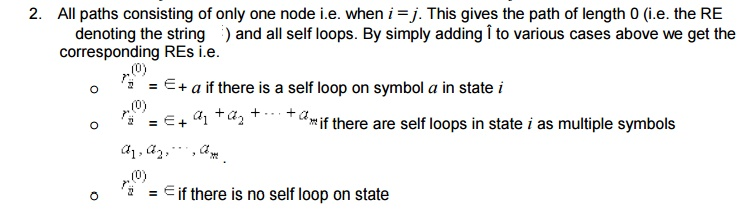Induction :

Assume that there exists a path from state i to state j such that there is no intermediate state whose number greater than k. The corresponding Re for the label of the path is  rij(k). There are only two possible cases :

1.       The path dose not go through the state k at all i.e. number of all the intermediate states are less than k. So, the label of the path from state i to state j is tha language described by the rij(k-1).

2.       The path goes through the state k at least once. The path may go from i to j and k may appear more than once. We can break the into pieces as shown in the figure 7.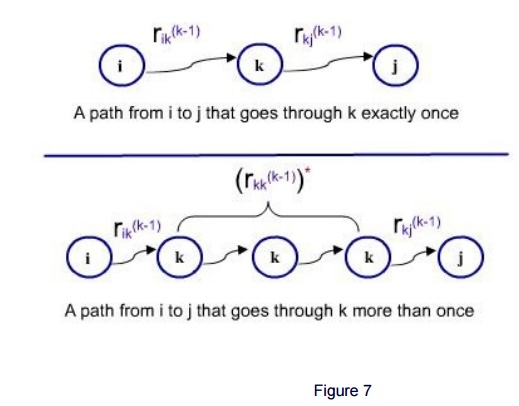1. The first part from the state i to the state k which is the first recurence. In this path, all intermediate states are less than k and it starts at iand ends at k. So the RE rik(k-1) denotes the language of the label of path.

2. The last part from the last occurence of the state k in the path to state j. In this path also, no intermediate state is numbered greater than k. Hence the RE  rkj(k-1) denoting the language of the label of the path.

3. In the middle, for the first occurence of k to the last occurence of k , represents a loop which may be taken zero times, once or any no of times. And all states between two consecutive k's are numbered less than k.

Hence the label of the path of the part is denoted by the RE rij(k-1)*. The label of the path from state i to state j is the concatenation of these 3 parts which isSince either case 1 or case 2 may happen the labels of all paths from state i to j is denoted by the followingSince  rij1(n) is the set of all strings that starts at start state 1 and finishes at final      following the transition of the FA with any value of the intermediate state (1, 2, ... , n) and hence accepted by the automata.

Regular Grammar: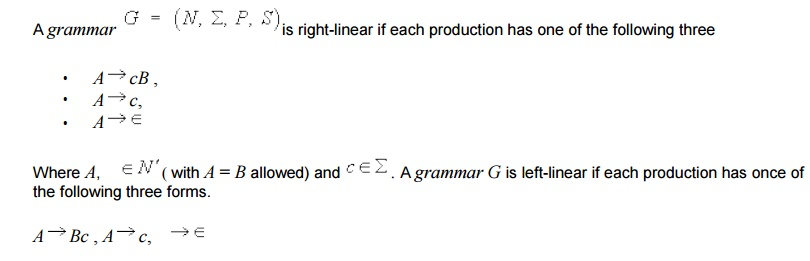A right or left-linear grammar is called a regular grammar.

Regular grammar and Finite Automata are equivalent as stated in the following

Theorem : A language L is regular iff it has a regular grammar. We use the following two lemmas to prove the above theorem.

Lemma 1 : If L is a regular language, then L is generated by some right-linearBy construction, the grammar G will have one production for each of the above transitions. Therefore, we have the corresponding derivation.then the derivation of w in G must have the form as given above. But, then the construction of G from M implies thatWe now show that this construction works.It is easy to see that G generates the language denoted by the regular expression (01)*0.

The construction of lemma 2 for this grammar produces the follwoing FA. This FA accepts exactly (01)*1.

Decisions Algorithms for CFL

In this section, we examine some questions about CFLs we can answer. A CFL may be represented using a CFG or PDA. But an algorithm that uses one representation can be made to work for the others, since we can construct one from the other.

Testing Emptiness :

Theorem : There are algorithms to test emptiness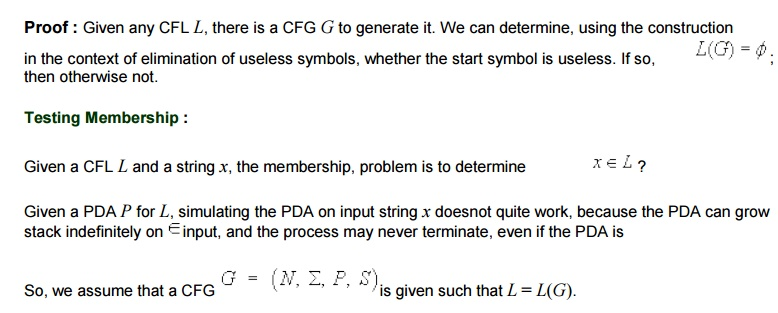Let us first present a simple but inefficient algorithm.Pumping Lemma:

Limitations of Finite Automata and Non regular Languages

The class of languages recognized by FA s is strictly the regular set. There are certain languages which are non regular i.e. cannot be recognized by any FAIn order to accept is language, we find that, an automaton seems to need to remember when passing the center point between a's and b's how many a's it has seen so far. Because it would have to compare that with the number of b's to either accept (when the two numbers are same) or reject (when they are not same) the But the number of a's is not limited and may be much larger than the number of states since the string may be arbitrarily long. So, the amount of information the automaton need to remember is unbounded.

A finite automaton cannot remember this with only finite memory (i.e. finite number of states). The fact that FA s have finite memory imposes some limitations on the structure of the languages recognized. Inductively, we can say that a language is regular only if in processing any string in this language, the information that has to be remembered at any point is strictly limited. The argument given above to show that  is non regular is informal. We now present a formal method for showing that anbn certain languages such anbn are non regular

Properties of CFL’s

Closure properties of CFL:

We consider some important closure properties of CFLs.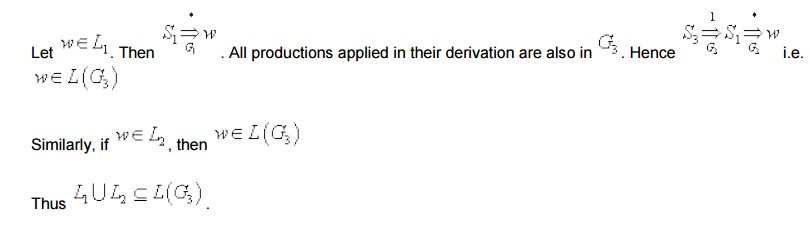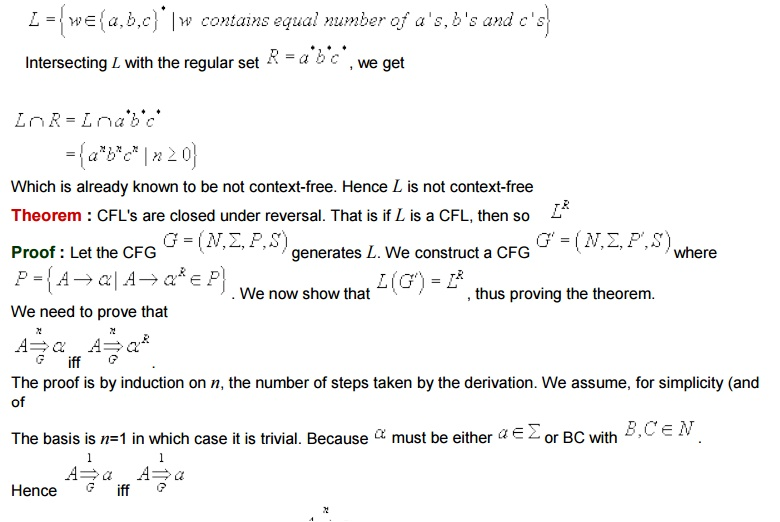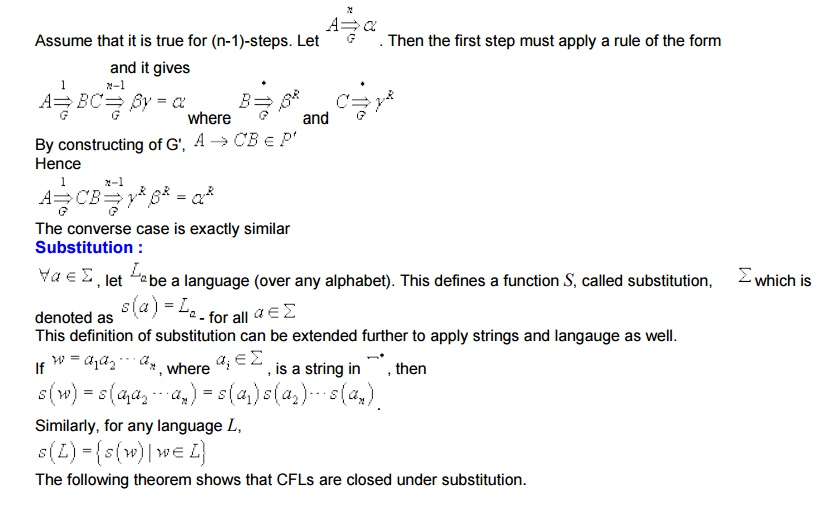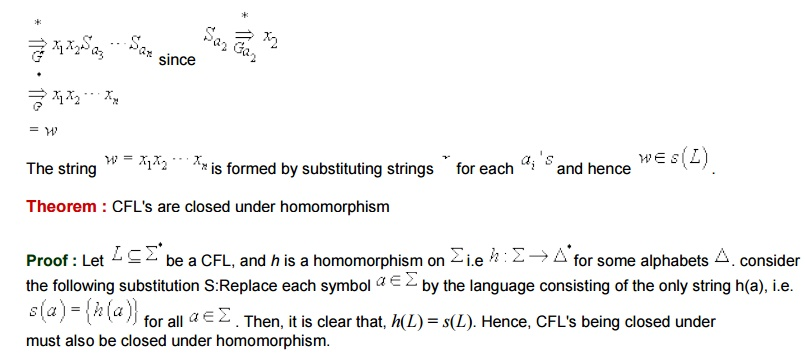Study Material, Lecturing Notes, Assignment, Reference, Wiki description explanation, brief detail
Theory of Computation : Grammars - Regular Expressions and Languages |